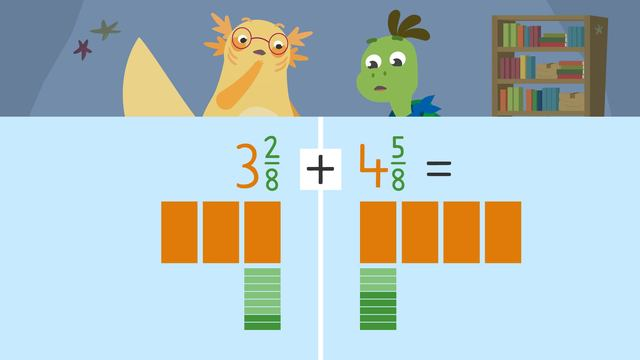# Adding and Subtracting Mixed NumbersRating

Ø 5.0 / 1 ratings

The authorsTeam Digital
CCSS.MATH.CONTENT.4.NF.B.3.C CCSS.MATH.CONTENT.4.NF.B.3.D

## Basics on the topicAdding and Subtracting Mixed Numbers

### How do you add and subtract mixed numbers?

Axel and Tank find a recipe, but they cannot tell what the title says. In order to figure out what the recipe makes, they need to practice adding and subtracting mixed numbers so they know how much of each ingredient to use.

#### Steps to Adding and Subtracting Mixed Numbers

When you practice adding and subtracting mixed numbers with like denominators, you can follow these three steps:

• Step One: Add or subtract the whole numbers.
• Step Two: Add or subtract the fractions.

#### Adding and Subtracting Mixed Numbers Examples

Let’s start by adding three and two-eighths and four and five-eighths.First, we need to find the sum of the whole numbers. That means, we are adding three and four first to get the sum seven.

Next, we find the sum of the fractions. Remember, when adding and subtracting fractions with common denominators we simply add or subtract the numerators and the denominators stay the same. Two-eighths plus five-eighths equals seven-eights.Our last step is to simplify our answer if we need to. A fraction is in simplest form when the numerator and denominator have no common factors other than one. Since seven and seven-eighths is in simplest form we can skip this step.

Now let’s practice subtracting mixed numbers by calculating five and three-fourths minus four and one-fourth.First, we need to find the difference of the whole numbers. That means, we are subtracting five and four first to get the difference of one.

Next, we find the difference of the fractions. Three-fourths minus one-fourth equals two-fourths.Our last step is to simplify our answer if we need to. The numerator and the denominator have the common factor of two, which means it can be simplified to one-half.#### How to Add and Subtract Mixed Numbers Summary

Remember, when you add and subtract mixed numbers with like denominators, you can follow these three steps:

• Step One: Add or subtract the whole numbers.
• Step Two: Add or subtract the fractions.